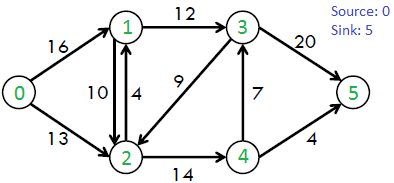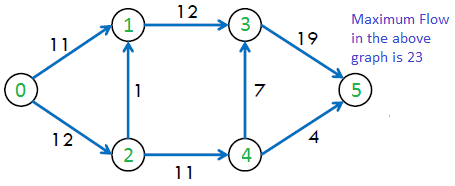# Push Relabel Algorithm | Set 2 (Implementation)

• Difficulty Level : Hard
• Last Updated : 08 Apr, 2022

We strongly recommend to refer below article before moving on to this article.
Push Relabel Algorithm | Set 1 (Introduction and Illustration)

Problem Statement: Given a graph that represents a flow network where every edge has a capacity. Also given two vertices source ‘s’ and sink ‘t’ in the graph, find the maximum possible flow from s to t with following constraints:
a) Flow on an edge doesn’t exceed the given capacity of the edge.
b) Incoming flow is equal to outgoing flow for every vertex except s and t.

For example, consider the following graph from CLRS book.The maximum possible flow in the above graph is 23.```Push-Relabel Algorithm
1) Initialize PreFlow : Initialize Flows and Heights

2) While it is possible to perform a Push() or Relabel() on a vertex
// Or while there is a vertex that has excess flow
Do Push() or Relabel()

// At this point all vertices have Excess Flow as 0 (Except source
// and sink)
3) Return flow.```

Below are main operations performed in Push Relabel algorithm.

There are three main operations in Push-Relabel Algorithm

1. Initialize PreFlow() It initializes heights and flows of all vertices.

```Preflow()
1) Initialize height and flow of every vertex as 0.
2) Initialize height of source vertex equal to total
number of vertices in graph.
3) Initialize flow of every edge as 0.
4) For all vertices adjacent to source s, flow and
excess flow is equal to capacity initially.```

2. Push() is used to make the flow from a node that has excess flow. If a vertex has excess flow and there is an adjacent with a smaller height (in the residual graph), we push the flow from the vertex to the adjacent with a lower height. The amount of pushed flow through the pipe (edge) is equal to the minimum of excess flow and capacity of the edge.

3. Relabel() operation is used when a vertex has excess flow and none of its adjacents is at the lower height. We basically increase the height of the vertex so that we can perform push(). To increase height, we pick the minimum height adjacent (in residual graph, i.e., an adjacent to whom we can add flow) and add 1 to it.

Implementation

The following implementation uses the below structure for representing a flow network.

```struct Vertex
{
int h;      // Height of node
int e_flow; // Excess Flow
}```
```struct Edge
{
int u, v; // Edge is from u to v
int flow; // Current flow
int capacity;
}```
```class Graph
{
Edge edge[];   // Array of edges
Vertex ver[];  // Array of vertices
}```

The below code uses the given graph itself as a flow network and residual graph. We have not created a separate graph for the residual graph and have used the same graph for simplicity.

## C++

 `// C++ program to implement push-relabel algorithm for``// getting maximum flow of graph``#include ``using` `namespace` `std;``  ` `struct` `Edge``{``    ``// To store current flow and capacity of edge``    ``int` `flow, capacity;``  ` `    ``// An edge u--->v has start vertex as u and end``    ``// vertex as v.``    ``int` `u, v;``  ` `    ``Edge(``int` `flow, ``int` `capacity, ``int` `u, ``int` `v)``    ``{``        ``this``->flow = flow;``        ``this``->capacity = capacity;``        ``this``->u = u;``        ``this``->v = v;``    ``}``};``  ` `// Represent a Vertex``struct` `Vertex``{``    ``int` `h, e_flow;``  ` `    ``Vertex(``int` `h, ``int` `e_flow)``    ``{``        ``this``->h = h;``        ``this``->e_flow = e_flow;``    ``}``};``  ` `// To represent a flow network``class` `Graph``{``    ``int` `V;    ``// No. of vertices``    ``vector ver;``    ``vector edge;``  ` `    ``// Function to push excess flow from u``    ``bool` `push(``int` `u);``  ` `    ``// Function to relabel a vertex u``    ``void` `relabel(``int` `u);``  ` `    ``// This function is called to initialize``    ``// preflow``    ``void` `preflow(``int` `s);``  ` `    ``// Function to reverse edge``    ``void` `updateReverseEdgeFlow(``int` `i, ``int` `flow);``  ` `public``:``    ``Graph(``int` `V);  ``// Constructor``  ` `    ``// function to add an edge to graph``    ``void` `addEdge(``int` `u, ``int` `v, ``int` `w);``  ` `    ``// returns maximum flow from s to t``    ``int` `getMaxFlow(``int` `s, ``int` `t);``};``  ` `Graph::Graph(``int` `V)``{``    ``this``->V = V;``  ` `    ``// all vertices are initialized with 0 height``    ``// and 0 excess flow``    ``for` `(``int` `i = 0; i < V; i++)``        ``ver.push_back(Vertex(0, 0));``}``  ` `void` `Graph::addEdge(``int` `u, ``int` `v, ``int` `capacity)``{``    ``// flow is initialized with 0 for all edge``    ``edge.push_back(Edge(0, capacity, u, v));``}``  ` `void` `Graph::preflow(``int` `s)``{``    ``// Making h of source Vertex equal to no. of vertices``    ``// Height of other vertices is 0.``    ``ver[s].h = ver.size();``  ` `    ``//``    ``for` `(``int` `i = 0; i < edge.size(); i++)``    ``{``        ``// If current edge goes from source``        ``if` `(edge[i].u == s)``        ``{``            ``// Flow is equal to capacity``            ``edge[i].flow = edge[i].capacity;``  ` `            ``// Initialize excess flow for adjacent v``            ``ver[edge[i].v].e_flow += edge[i].flow;``  ` `            ``// Add an edge from v to s in residual graph with``            ``// capacity equal to 0``            ``edge.push_back(Edge(-edge[i].flow, 0, edge[i].v, s));``        ``}``    ``}``}``  ` `// returns index of overflowing Vertex``int` `overFlowVertex(vector& ver)``{``    ``for` `(``int` `i = 1; i < ver.size() - 1; i++)``       ``if` `(ver[i].e_flow > 0)``            ``return` `i;``  ` `    ``// -1 if no overflowing Vertex``    ``return` `-1;``}``  ` `// Update reverse flow for flow added on ith Edge``void` `Graph::updateReverseEdgeFlow(``int` `i, ``int` `flow)``{``    ``int` `u = edge[i].v, v = edge[i].u;``  ` `    ``for` `(``int` `j = 0; j < edge.size(); j++)``    ``{``        ``if` `(edge[j].v == v && edge[j].u == u)``        ``{``            ``edge[j].flow -= flow;``            ``return``;``        ``}``    ``}``  ` `    ``// adding reverse Edge in residual graph``    ``Edge e = Edge(0, flow, u, v);``    ``edge.push_back(e);``}``  ` `// To push flow from overflowing vertex u``bool` `Graph::push(``int` `u)``{``    ``// Traverse through all edges to find an adjacent (of u)``    ``// to which flow can be pushed``    ``for` `(``int` `i = 0; i < edge.size(); i++)``    ``{``        ``// Checks u of current edge is same as given``        ``// overflowing vertex``        ``if` `(edge[i].u == u)``        ``{``            ``// if flow is equal to capacity then no push``            ``// is possible``            ``if` `(edge[i].flow == edge[i].capacity)``                ``continue``;``  ` `            ``// Push is only possible if height of adjacent``            ``// is smaller than height of overflowing vertex``            ``if` `(ver[u].h > ver[edge[i].v].h)``            ``{``                ``// Flow to be pushed is equal to minimum of``                ``// remaining flow on edge and excess flow.``                ``int` `flow = min(edge[i].capacity - edge[i].flow,``                               ``ver[u].e_flow);``  ` `                ``// Reduce excess flow for overflowing vertex``                ``ver[u].e_flow -= flow;``  ` `                ``// Increase excess flow for adjacent``                ``ver[edge[i].v].e_flow += flow;``  ` `                ``// Add residual flow (With capacity 0 and negative``                ``// flow)``                ``edge[i].flow += flow;``  ` `                ``updateReverseEdgeFlow(i, flow);``  ` `                ``return` `true``;``            ``}``        ``}``    ``}``    ``return` `false``;``}``  ` `// function to relabel vertex u``void` `Graph::relabel(``int` `u)``{``    ``// Initialize minimum height of an adjacent``    ``int` `mh = INT_MAX;``  ` `    ``// Find the adjacent with minimum height``    ``for` `(``int` `i = 0; i < edge.size(); i++)``    ``{``        ``if` `(edge[i].u == u)``        ``{``            ``// if flow is equal to capacity then no``            ``// relabeling``            ``if` `(edge[i].flow == edge[i].capacity)``                ``continue``;``  ` `            ``// Update minimum height``            ``if` `(ver[edge[i].v].h < mh)``            ``{``                ``mh = ver[edge[i].v].h;``  ` `                ``// updating height of u``                ``ver[u].h = mh + 1;``            ``}``        ``}``    ``}``}``  ` `// main function for printing maximum flow of graph``int` `Graph::getMaxFlow(``int` `s, ``int` `t)``{``    ``preflow(s);``  ` `    ``// loop until none of the Vertex is in overflow``    ``while` `(overFlowVertex(ver) != -1)``    ``{``        ``int` `u = overFlowVertex(ver);``        ``if` `(!push(u))``            ``relabel(u);``    ``}``  ` `    ``// ver.back() returns last Vertex, whose``    ``// e_flow will be final maximum flow``    ``return` `ver.back().e_flow;``}``  ` `// Driver program to test above functions``int` `main()``{``    ``int` `V = 6;``    ``Graph g(V);``  ` `    ``// Creating above shown flow network``    ``g.addEdge(0, 1, 16);``    ``g.addEdge(0, 2, 13);``    ``g.addEdge(1, 2, 10);``    ``g.addEdge(2, 1, 4);``    ``g.addEdge(1, 3, 12);``    ``g.addEdge(2, 4, 14);``    ``g.addEdge(3, 2, 9);``    ``g.addEdge(3, 5, 20);``    ``g.addEdge(4, 3, 7);``    ``g.addEdge(4, 5, 4);``  ` `    ``// Initialize source and sink``    ``int` `s = 0, t = 5;``  ` `    ``cout << ``"Maximum flow is "` `<< g.getMaxFlow(s, t);``    ``return` `0;``}`

Output:

`Maximum flow is 23`